• +91 9971497814
• info@interviewmaterial.com

# RD Chapter 19- Surface Areas and Volume of a Circular Cylinder Ex-19.2 Interview Questions Answers

### Related Subjects

Question 1 :
A soft drink is available in two packs – (i) a tin can with a rectangular base of length 5 cm and width 4 cm, having a height of 15 cm and
(ii) a plastic cylinder with circular base of diameter 7 cm and height 10 cm. Which container has greater capacity and by how much? [NCERT]

Answer 1 : In first case, in a rectangular container of softdrink, the length of base = 5 cm
and Width = 4 cm
Height = 15 cm
Volume of soft drink = lbh= 5 x 4 x 15 = 300 cm3
and in second case, in a cylindrical container, diameter of base = 7 cm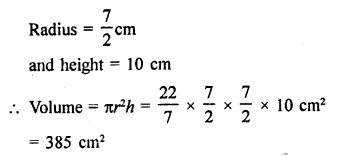Thesoft drink in second container is greater and how much greater = 385 cm – 380cm2 = 85 cm2

Question 2 : The pillars of a temple are cylindrically shaped. If each pillar has a circular base of radius 20 cm and height 10 m. How much concrete mixture would be required to build 14 such pillars? [NCERT]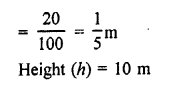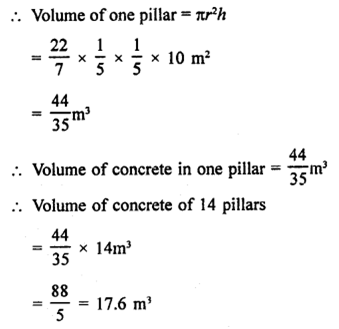Question 3 : The inner diameter of a cylindrical wooden pipe is 24cm and its outer diameter is 28 cm. The length of the pipe is 35 cm. Find themass of the pipe, if 1 cm3 of wood has a mass of 0.6 gm.[NCERT]

Answer 3 : Inner diameter of a cylindrical wooden pipe = 24 cm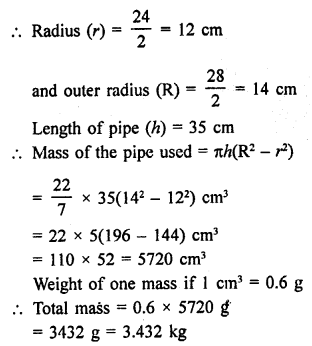Question 4 : If the lateral surface of a cylinder is 94.2 cm2 andits height is 5 cm, find:
(ii) volume of the cylinder [Use π = 3.14] [NCERT]

Answer 4 : Lateral surface area of a cylinder = 94.2 cm2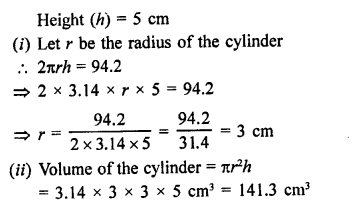Question 5 : The capacity of a closed cylindrical vessel of height1 m is 15.4 litres. How many square metres of metal sheet would be needed tomake it? [NCERT]

Answer 5 : The capacity of a closed cylindrical vessel = 15.4 l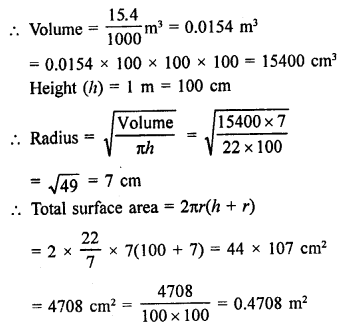Question 6 : A patient in a hospital is given soup daily in acylindrical bowl of diameter 7 cm. If the bowl is filled with soup to a heightof 4 cm, how much soup the hospital has to prepare daily to serve 250 patients?[NCERT]

Answer 6 : Diameter of the cylindrical bowl = 7 cm
Level of soup in it = 4 cm
Volume of soup in one bowlfor one patient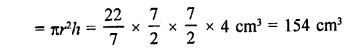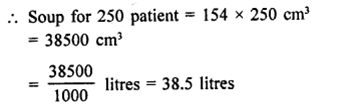Question 7 : A hollow garden roller, 63 cm wide with a girth of 440cm, is made of 4 cm thick iron. Find the volume of the iron.

Answer 7 : Width of hollow cylinder (A) = 63 cm
Girth = 440 cm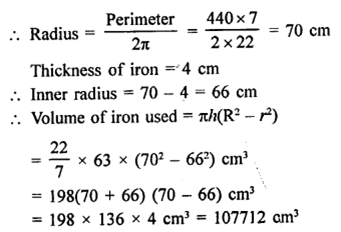Question 8 : The cost of painting the total outside surface of aclosed cylindrical oil tank at 50 paise per square decimetre is ₹ 198. Theheight of the tank is 6 times the radius of the base of the tank. Find thevolume corrected to 2 decimal places.

Answer 8 : Rate of painting = 50 paise per dm2
Total cost = ₹198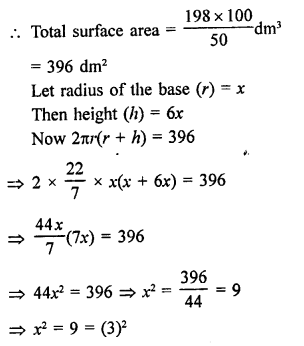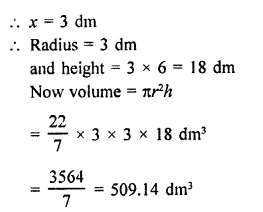Question 9 : The radii of two cylinders are in the ratio 2 : 3 andtheir heights are in the ratio 5:3. Calculate the ratio of their volumes andthe ratio of their curved surfaces.

Ratio in radii of two cylinders = 2:3
and ratio in their heights = 5:3
Let radius of the first cylinder (r1) = 2x
and radius of second cylinder (r2) = 3x
and height of first cylinders (h1) = 5y
and height of second cylinder (h2) = 3y
(i) Now volume of the first cylinder = πr2h = π(2x)2 x5y = 20πx22y
and volume of tlie second cylinder = π(3x)2 x 3y = π x 9×2 x 3y= 27πx2y
Now ratio in their volume
= 20πx2y : 21πx2y = 20 : 27
(ii) Curved surface area of first cylinder = 2πrh = 2π x 2x x 5y =20πxy
and curved surface area of second cylinder = 2π x 3x x = 1 8πxy
Ratioin their curved surface area
= 20πxy : 18πxy = 10 : 9

Question 10 : The ratio between the curved surface area and the total surface area of a right circular cylinder is 1 : 2. Find the volume of the cylinder, if its total surface area is 616 cm2.

Answer 10 : Ratio in curved surface area and total surface area ofa cylinder =1:2
Total surface area = 616 cm2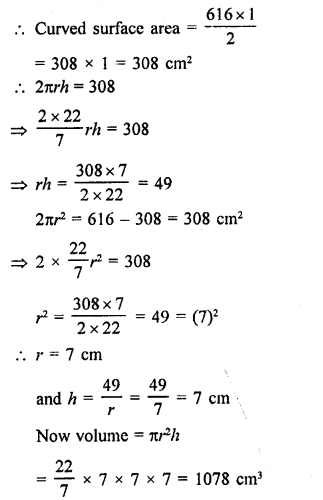Todays Deals### RD Chapter 19- Surface Areas and Volume of a Circular Cylinder Ex-19.2 Contributorskrishan

Name:
Email:

# Latest News# 9000 interview questions in different categories